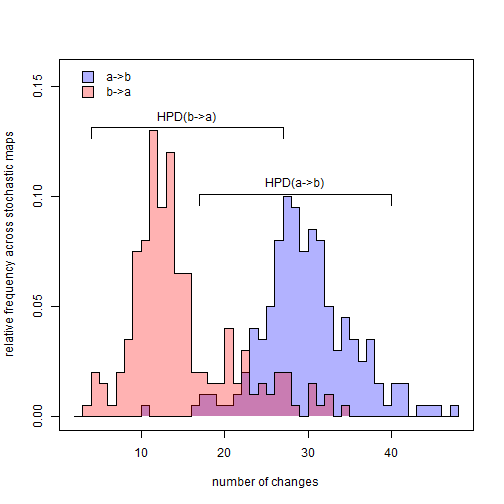## Saturday, October 29, 2016

### Testing hypotheses about the number of changes of different types on the tree using stochastic mapping

A phytools user recently asked me the following:

“I have been using the function `countSimmap` to count the transitions between short snouts and long snouts. However, I cannot figure out how to statistically test if there have been significantly more transitions to one state than the other. Is there a function for this in phytools?”

Well, stochastic character mapping is a Bayesian method - so we can't exactly test for 'significance', per se. However, we could, for example, sample transitions matrices (Q) and character histories from their posterior distribution, and then ask whether HPD intervals from the two overlap. We can even do some other cool stuff, like visualize the posterior probability distribution of changes across the two trees.

Here's a quick example with some simulated data:

``````library(phytools)
tree
``````
``````##
## Phylogenetic tree with 200 tips and 199 internal nodes.
##
## Tip labels:
##  t185, t186, t168, t146, t187, t188, ...
##
## Rooted; includes branch lengths.
``````
``````x
``````
``````## t185 t186 t168 t146 t187 t188 t111  t59  t60  t36  t27  t19  t20 t117 t118
##    b    b    a    a    b    b    b    a    b    b    b    a    a    b    a
## t103  t82 t123 t124  t28 t167 t195 t196 t126 t177 t178 t153 t154  t49 t147
##    a    a    b    b    a    a    a    a    a    a    a    a    a    a    b
## t148 t136 t137 t108 t109 t144 t145  t77  t78   t8   t9 t142 t143 t104 t105
##    b    b    b    a    a    b    b    b    b    b    a    b    b    b    b
## t175 t176  t45  t64 t115 t116 t157 t158   t5  t57  t58  t53  t47  t65 t149
##    b    b    b    b    b    b    b    b    b    b    b    b    b    a    b
## t150 t113  t91  t51  t52 t119 t120  t10  t11  t66  t67  t46  t15  t23  t95
##    a    a    b    a    b    a    a    a    a    b    a    b    a    b    b
##  t96  t99 t100  t43  t16  t62  t63  t86  t87 t181 t182  t84 t101 t102  t68
##    b    a    a    a    b    a    b    b    b    b    b    b    b    b    b
## t112 t155 t156  t70  t21  t50 t169 t170  t61 t110 t189 t190  t13  t85  t89
##    b    b    b    b    b    a    a    a    a    a    a    a    a    a    a
##  t90  t40 t165 t166  t17  t18  t34  t97  t98  t72  t73 t173 t174  t48  t30
##    a    b    b    b    a    a    b    b    b    a    a    a    a    b    a
##  t69 t127 t128  t81 t179 t180 t159 t160  t29  t37  t38 t199 t200 t163 t164
##    b    b    b    b    a    a    a    a    a    b    b    a    a    a    a
##   t7  t22  t44  t92 t131 t132  t74 t191 t192 t114  t12  t31  t32  t41  t42
##    a    b    a    b    b    b    b    b    b    b    b    b    b    b    b
##   t4  t14  t33  t55 t193 t194  t26  t54  t79  t80 t106 t107 t138 t139 t133
##    b    b    b    b    b    b    b    b    b    b    b    b    a    a    a
##  t39  t75  t76   t6  t83 t140 t141  t56  t24  t25  t71 t171 t172 t134 t135
##    a    a    a    b    a    a    a    a    b    b    b    b    b    b    b
## t125 t183 t184  t93  t94 t121 t122  t35   t2 t197 t198  t88 t129 t130 t161
##    b    b    b    b    b    b    b    b    b    b    b    b    b    b    b
## t162   t3 t151 t152   t1
##    b    b    b    b    a
## Levels: a b
``````
``````trees<-make.simmap(tree,x,model="ARD",Q="mcmc",nsim=200)
``````
``````## Running MCMC burn-in. Please wait....
## Running 20000 generations of MCMC, sampling every 100 generations.
##
## make.simmap is simulating with a sample of Q from
## the posterior distribution
##
## Mean Q from the posterior is
## Q =
##            a          b
## a -1.4479062  1.4479062
## b  0.5834969 -0.5834969
## and (mean) root node prior probabilities
## pi =
##  0.5 0.5
``````
``````## Done.
``````
``````obj<-summary(trees)
ab<-obj\$count[,2]
ba<-obj\$count[,3]
class(ab)<-class(ba)<-"mcmc"
library(coda)
hpd.ab<-HPDinterval(ab)
hpd.ba<-HPDinterval(ba)
hpd.ab
``````
``````##      lower upper
## var1    17    40
## attr(,"Probability")
##  0.95
``````
``````hpd.ba
``````
``````##      lower upper
## var1     4    27
## attr(,"Probability")
##  0.95
``````

We can see that in this case at least the posterior distributions broadly overlap.

Let's go a step further, and visualize these distributions:

``````p.ab<-hist(ab,breaks=seq(min(c(ab,ba))-1.5,
max(c(ab,ba))+1.5,1),plot=FALSE)
p.ba<-hist(ba,breaks=seq(min(c(ab,ba))-1.5,
max(c(ab,ba))+1.5,1),plot=FALSE)
plot(p.ab\$mids,p.ab\$density,xlim=c(min(c(ab,ba))-1,
max(c(ab,ba))+1),ylim=c(0,1.2*max(c(p.ab\$density,
p.ba\$density))),
type="n",xlab="number of changes",
ylab="relative frequency across stochastic maps")
y2<-rep(p.ab\$density,each=2)
y2<-y2[-length(y2)]
x2<-rep(p.ab\$mids,each=2)[-1]
x3<-c(min(x2),x2,max(x2))
y3<-c(0,y2,0)
polygon(x3,y3,col=make.transparent("blue",0.3),border=FALSE)
lines(p.ab\$mids,p.ab\$density,type="s")
y2<-rep(p.ba\$density,each=2)
y2<-y2[-length(y2)]
x2<-rep(p.ba\$mids,each=2)[-1]
x3<-c(min(x2),x2,max(x2))
y3<-c(0,y2,0)
polygon(x3,y3,col=make.transparent("red",0.3),border=FALSE)
lines(p.ba\$mids,p.ba\$density,type="s")
make.transparent("red",0.3)),c("a?b","b?a")),
prompt=FALSE,x=min(c(ab,ba)),y=0.95*par()\$usr)
lines(hpd.ab,rep(1.01*max(p.ab\$density),2))
lines(rep(hpd.ab,2),c(1.01*max(p.ab\$density),
1.01*max(p.ab\$density)-0.005))
lines(rep(hpd.ab,2),c(1.01*max(p.ab\$density),
1.01*max(p.ab\$density)-0.005))
text(mean(hpd.ab),1.01*max(p.ab\$density),"HPD(a?b)",
pos=3)
lines(hpd.ba,rep(1.01*max(p.ba\$density),2))
lines(rep(hpd.ba,2),c(1.01*max(p.ba\$density),
1.01*max(p.ba\$density)-0.005))
lines(rep(hpd.ba,2),c(1.01*max(p.ba\$density),
1.01*max(p.ba\$density)-0.005))
text(mean(hpd.ba),1.01*max(p.ba\$density),"HPD(b?a)",
pos=3)
``````Pretty cool right?

Note that in a likelihood framework we can look at the problem a different way - say by testing the null hypothesis that backward & forward rates are equal, or that one or the other rate is zero.

Finally, the data for this exercise were simulated as follows:

``````Q<-matrix(c(-1.2,1.2,0.5,-0.5),2,2,byrow=TRUE)
rownames(Q)<-colnames(Q)<-c("a","b")
tree<-pbtree(n=200,scale=1)
x<-sim.history(tree,Q,anc="a")\$states
x<-as.factor(x)
``````

#### 1 comment:

1.Excelente!!!! Muchas Gracias!!!

Note: due to the very large amount of spam, all comments are now automatically submitted for moderation.